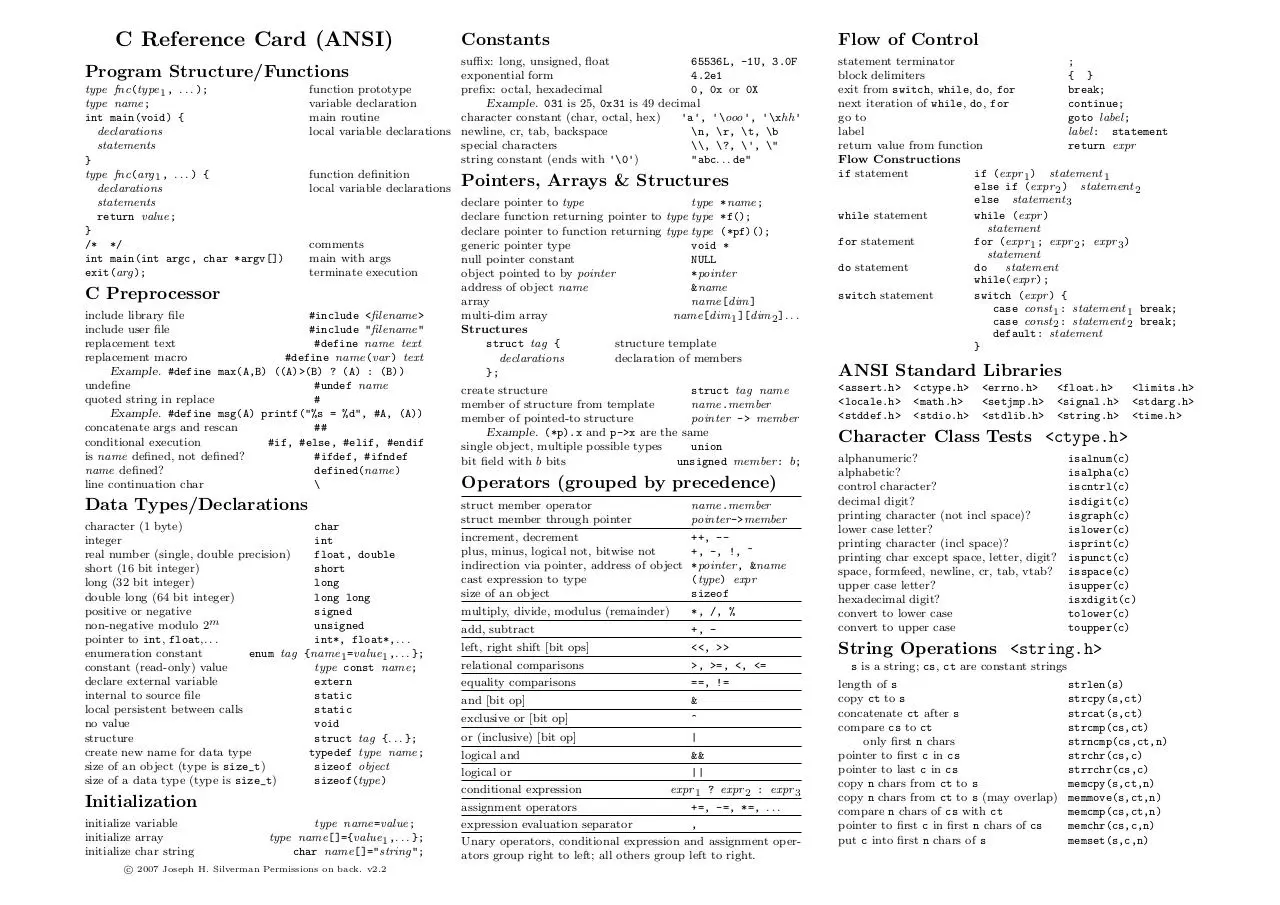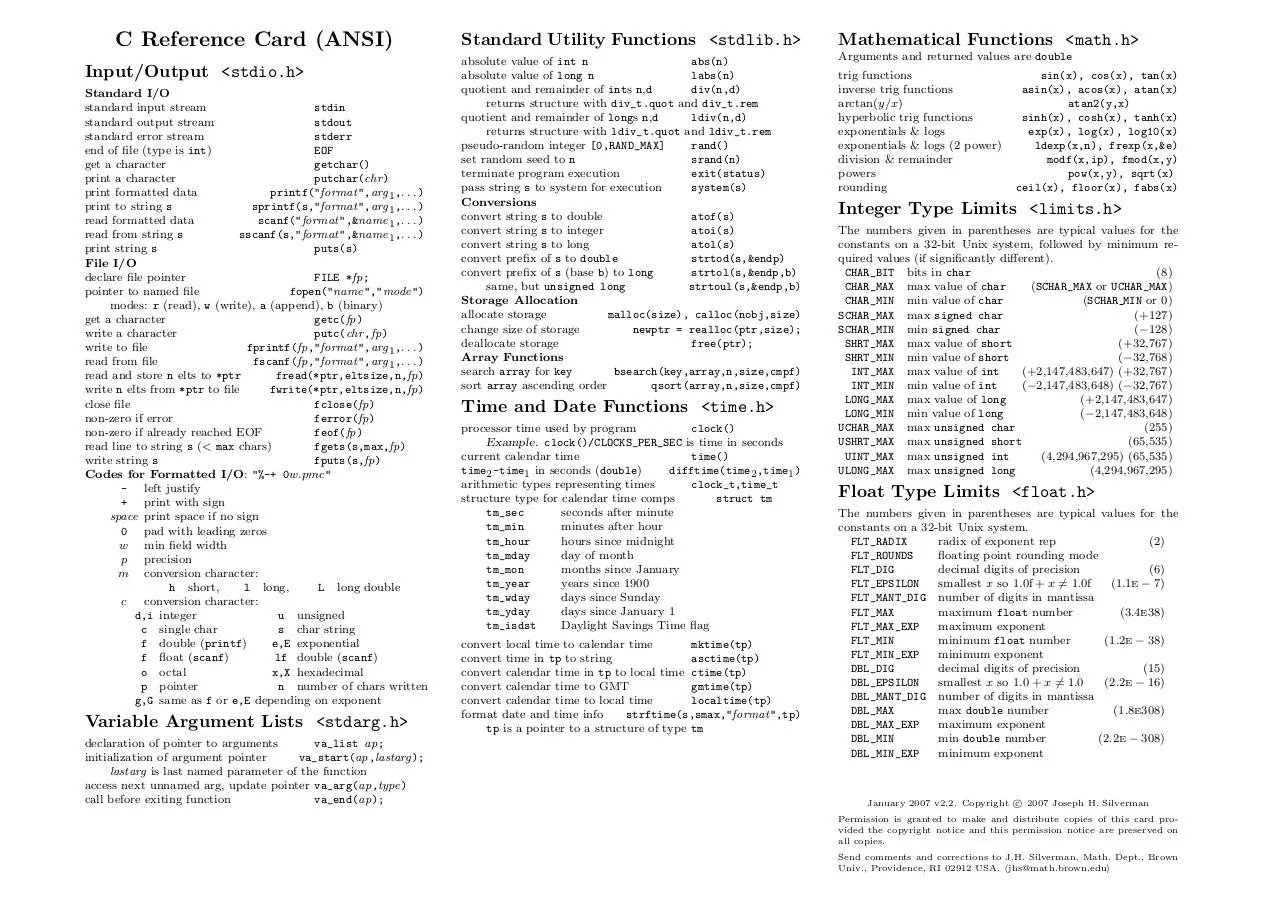# CRefCard.v2.2 .pdf

### File information

This PDF 1.2 document has been generated by TeX output 2007.01.17:1623 / dvipdfm 0.13.2c, Copyright Â© 1998, by Mark A. Wicks, and has been sent on pdf-archive.com on 06/04/2015 at 03:07, from IP address 93.192.x.x. The current document download page has been viewed 1556 times.
File size: 105.75 KB (2 pages).
Privacy: public file

### Document preview

C Reference Card (ANSI)

Constants

Flow of Control

suffix: long, unsigned, float
65536L, -1U, 3.0F
Program Structure/Functions
exponential form
4.2e1
0, 0x or 0X
type fnc(type 1 , . . . );
function prototype
Example. 031 is 25, 0x31 is 49 decimal
type name;
variable declaration
character constant (char, octal, hex)
'a', '\ooo', '\xhh'
int main(void) {
main routine
\n, \r, \t, \b
declarations
local variable declarations newline, cr, tab, backspace
special characters
\\, \?, \', \"
statements
string constant (ends with '\0')
"abc. . . de"
}
type fnc(arg 1 , . . . ) {
function definition
declarations
local variable declarations Pointers, Arrays &amp; Structures
statements
declare pointer to type
type *name;
return value;
declare function returning pointer to type type *f();
}
declare pointer to function returning type type (*pf)();
/* */
generic pointer type
void *
int main(int argc, char *argv[])
main with args
null pointer constant
NULL
exit(arg);
terminate execution
object pointed to by pointer
*pointer
&amp;name
C Preprocessor
array
name[dim]
include library file
#include &lt;filename&gt;
multi-dim array
name[dim 1 ][dim 2 ]. . .
include user file
#include "filename"
Structures
replacement text
#define name text
struct tag {
structure template
replacement macro
#define name(var ) text
declarations
declaration of members
Example. #define max(A,B) ((A)&gt;(B) ? (A) : (B))
};
undefine
#undef name
create structure
struct tag name
quoted string in replace
#
member of structure from template
name.member
Example. #define msg(A) printf("%s = %d", #A, (A))
member of pointed-to structure
pointer -&gt; member
concatenate args and rescan
##
Example. (*p).x and p-&gt;x are the same
conditional execution
#if, #else, #elif, #endif
single object, multiple possible types
union
is name defined, not defined?
#ifdef, #ifndef
bit field with b bits
unsigned member : b;
name defined?
defined(name)
line continuation char
\
Operators (grouped by precedence)

Data Types/Declarations
character (1 byte)
char
integer
int
real number (single, double precision)
float, double
short (16 bit integer)
short
long (32 bit integer)
long
double long (64 bit integer)
long long
positive or negative
signed
non-negative modulo 2m
unsigned
pointer to int, float,. . .
int*, float*,. . .
enumeration constant
enum tag {name 1 =value 1 ,. . . };
type const name;
declare external variable
extern
internal to source file
static
local persistent between calls
static
no value
void
structure
struct tag {. . . };
create new name for data type
typedef type name;
size of an object (type is size_t)
sizeof object
size of a data type (type is size_t)
sizeof(type)

Initialization
initialize variable
initialize array
initialize char string

type name=value;
type name[]={value 1 ,. . . };
char name[]="string";

c 2007 Joseph H. Silverman Permissions on back. v2.2
°

statement terminator
;
block delimiters
{ }
exit from switch, while, do, for
break;
next iteration of while, do, for
continue;
go to
goto label;
label
label: statement
return value from function
return expr
Flow Constructions
if statement
if (expr 1 ) statement 1
else if (expr 2 ) statement 2
else statement 3
while statement
while (expr )
statement
for statement
for (expr 1 ; expr 2 ; expr 3 )
statement
do statement
do
statement
while(expr );
switch statement
switch (expr ) {
case const 1 : statement 1 break;
case const 2 : statement 2 break;
default: statement
}

ANSI Standard Libraries
&lt;assert.h&gt;
&lt;locale.h&gt;
&lt;stddef.h&gt;

&lt;ctype.h&gt;
&lt;math.h&gt;
&lt;stdio.h&gt;

&lt;errno.h&gt;
&lt;setjmp.h&gt;
&lt;stdlib.h&gt;

&lt;float.h&gt;
&lt;signal.h&gt;
&lt;string.h&gt;

Character Class Tests &lt;ctype.h&gt;

struct member operator
struct member through pointer
increment, decrement
plus, minus, logical not, bitwise not
indirection via pointer, address of object
cast expression to type
size of an object

name.member
pointer -&gt;member
++, -+, -, !, ~
*pointer , &amp;name
(type) expr
sizeof

multiply, divide, modulus (remainder)

*, /, %

+, -

alphanumeric?
alphabetic?
control character?
decimal digit?
printing character (not incl space)?
lower case letter?
printing character (incl space)?
printing char except space, letter, digit?
space, formfeed, newline, cr, tab, vtab?
upper case letter?
convert to lower case
convert to upper case

left, right shift [bit ops]

&lt;&lt;, &gt;&gt;

String Operations &lt;string.h&gt;

relational comparisons
equality comparisons

&gt;, &gt;=, &lt;, &lt;=
==, !=

and [bit op]

&amp;

exclusive or [bit op]

^

or (inclusive) [bit op]

|

logical and
&amp;&amp;
logical or
||
conditional expression
expr 1 ? expr 2 : expr 3
assignment operators
+=, -=, *=, . . .
expression evaluation separator
,
Unary operators, conditional expression and assignment operators group right to left; all others group left to right.

&lt;limits.h&gt;
&lt;stdarg.h&gt;
&lt;time.h&gt;

isalnum(c)
isalpha(c)
iscntrl(c)
isdigit(c)
isgraph(c)
islower(c)
isprint(c)
ispunct(c)
isspace(c)
isupper(c)
isxdigit(c)
tolower(c)
toupper(c)

s is a string; cs, ct are constant strings
length of s
copy ct to s
concatenate ct after s
compare cs to ct
only first n chars
pointer to first c in cs
pointer to last c in cs
copy n chars from ct to s
copy n chars from ct to s (may overlap)
compare n chars of cs with ct
pointer to first c in first n chars of cs
put c into first n chars of s

strlen(s)
strcpy(s,ct)
strcat(s,ct)
strcmp(cs,ct)
strncmp(cs,ct,n)
strchr(cs,c)
strrchr(cs,c)
memcpy(s,ct,n)
memmove(s,ct,n)
memcmp(cs,ct,n)
memchr(cs,c,n)
memset(s,c,n)

C Reference Card (ANSI)
Input/Output &lt;stdio.h&gt;
Standard I/O
standard input stream
stdin
standard output stream
stdout
standard error stream
stderr
end of file (type is int)
EOF
get a character
getchar()
print a character
putchar(chr )
print formatted data
printf("format",arg 1 ,. . . )
print to string s
sprintf(s,"format",arg 1 ,. . . )
scanf("format",&amp;name 1 ,. . . )
sscanf(s,"format",&amp;name 1 ,. . . )
print string s
puts(s)
File I/O
declare file pointer
FILE *fp;
pointer to named file
fopen("name","mode")
modes: r (read), w (write), a (append), b (binary)
get a character
getc(fp)
write a character
putc(chr ,fp)
write to file
fprintf(fp,"format",arg 1 ,. . . )
fscanf(fp,"format",arg 1 ,. . . )
read and store n elts to *ptr
write n elts from *ptr to file
fwrite(*ptr,eltsize,n,fp)
close file
fclose(fp)
non-zero if error
ferror(fp)
feof(fp)
read line to string s (&lt; max chars)
fgets(s,max,fp)
write string s
fputs(s,fp)
Codes for Formatted I/O: "%-+ 0w.pmc"
- left justify
+ print with sign
space print space if no sign
w min field width
p precision
m conversion character:
h short,
l long,
L long double
c
conversion character:
d,i integer
u unsigned
c single char
s char string
f double (printf)
e,E exponential
f float (scanf)
lf double (scanf)
o octal
p pointer
n number of chars written
g,G same as f or e,E depending on exponent

Variable Argument Lists &lt;stdarg.h&gt;
declaration of pointer to arguments
va_list ap;
initialization of argument pointer
va_start(ap,lastarg);
lastarg is last named parameter of the function
access next unnamed arg, update pointer va_arg(ap,type)
call before exiting function
va_end(ap);

Standard Utility Functions &lt;stdlib.h&gt;
absolute value of int n
abs(n)
absolute value of long n
labs(n)
quotient and remainder of ints n,d
div(n,d)
returns structure with div_t.quot and div_t.rem
quotient and remainder of longs n,d
ldiv(n,d)
returns structure with ldiv_t.quot and ldiv_t.rem
pseudo-random integer [0,RAND_MAX]
rand()
set random seed to n
srand(n)
terminate program execution
exit(status)
pass string s to system for execution
system(s)
Conversions
convert string s to double
atof(s)
convert string s to integer
atoi(s)
convert string s to long
atol(s)
convert prefix of s to double
strtod(s,&amp;endp)
convert prefix of s (base b) to long
strtol(s,&amp;endp,b)
same, but unsigned long
strtoul(s,&amp;endp,b)
Storage Allocation
allocate storage
malloc(size), calloc(nobj,size)
change size of storage
newptr = realloc(ptr,size);
deallocate storage
free(ptr);
Array Functions
search array for key
bsearch(key,array,n,size,cmpf)
sort array ascending order
qsort(array,n,size,cmpf)

Time and Date Functions &lt;time.h&gt;
processor time used by program
clock()
Example. clock()/CLOCKS_PER_SEC is time in seconds
current calendar time
time()
time2 -time1 in seconds (double)
difftime(time2 ,time1 )
arithmetic types representing times
clock_t,time_t
structure type for calendar time comps
struct tm
tm_sec
seconds after minute
tm_min
minutes after hour
tm_hour
hours since midnight
tm_mday
day of month
tm_mon
months since January
tm_year
years since 1900
tm_wday
days since Sunday
tm_yday
days since January 1
tm_isdst
Daylight Savings Time flag
convert local time to calendar time
mktime(tp)
convert time in tp to string
asctime(tp)
convert calendar time in tp to local time ctime(tp)
convert calendar time to GMT
gmtime(tp)
convert calendar time to local time
localtime(tp)
format date and time info
strftime(s,smax,"format",tp)
tp is a pointer to a structure of type tm

Mathematical Functions &lt;math.h&gt;
Arguments and returned values are double
trig functions
inverse trig functions
arctan(y/x)
hyperbolic trig functions
exponentials &amp; logs
exponentials &amp; logs (2 power)
division &amp; remainder
powers
rounding

sin(x), cos(x), tan(x)
asin(x), acos(x), atan(x)
atan2(y,x)
sinh(x), cosh(x), tanh(x)
exp(x), log(x), log10(x)
ldexp(x,n), frexp(x,&amp;e)
modf(x,ip), fmod(x,y)
pow(x,y), sqrt(x)
ceil(x), floor(x), fabs(x)

Integer Type Limits &lt;limits.h&gt;
The numbers given in parentheses are typical values for the
constants on a 32-bit Unix system, followed by minimum required values (if significantly different).
CHAR_BIT bits in char
(8)
CHAR_MAX max value of char
(SCHAR_MAX or UCHAR_MAX)
CHAR_MIN min value of char
(SCHAR MIN or 0)
SCHAR_MAX max signed char
(+127)
SCHAR_MIN min signed char
(−128)
SHRT_MAX max value of short
(+32,767)
SHRT_MIN min value of short
(−32,768)
INT_MAX max value of int
(+2,147,483,647) (+32,767)
INT_MIN min value of int
(−2,147,483,648) (−32,767)
LONG_MAX max value of long
(+2,147,483,647)
LONG_MIN min value of long
(−2,147,483,648)
UCHAR_MAX max unsigned char
(255)
USHRT_MAX max unsigned short
(65,535)
UINT_MAX max unsigned int
(4,294,967,295) (65,535)
ULONG_MAX max unsigned long
(4,294,967,295)

Float Type Limits &lt;float.h&gt;
The numbers given in parentheses are typical values for the
constants on a 32-bit Unix system.
(2)
FLT_ROUNDS
floating point rounding mode
FLT_DIG
decimal digits of precision
(6)
FLT_EPSILON
smallest x so 1.0f + x 6= 1.0f
(1.1E − 7)
FLT_MANT_DIG number of digits in mantissa
FLT_MAX
maximum float number
(3.4E38)
FLT_MAX_EXP
maximum exponent
FLT_MIN
minimum float number
(1.2E − 38)
FLT_MIN_EXP
minimum exponent
DBL_DIG
decimal digits of precision
(15)
DBL_EPSILON
smallest x so 1.0 + x 6= 1.0
(2.2E − 16)
DBL_MANT_DIG number of digits in mantissa
DBL_MAX
max double number
(1.8E308)
DBL_MAX_EXP
maximum exponent
DBL_MIN
min double number
(2.2E − 308)
DBL_MIN_EXP
minimum exponent

c 2007 Joseph H. Silverman
Permission is granted to make and distribute copies of this card provided the copyright notice and this permission notice are preserved on
all copies.
Send comments and corrections to J.H. Silverman, Math. Dept., Brown
Univ., Providence, RI 02912 USA. hjhs@math.brown.eduiCRefCard.v2.2.pdf (PDF, 105.75 KB)

### Share on social networks

#### HTML Code

Copy the following HTML code to share your document on a Website or Blog